Calculation of Flow Rate - Intergraph Smart Instrumentation - 13.1 - Reference - Hexagon PPM

Integraph Smart Instrumentation Sizing Equations

Language
English (United States)
Product
Intergraph Smart Instrumentation
Search by Category
Reference
Smart Instrumentation Version
13.1
1. Calculate internal pipe diameter at floe temperature: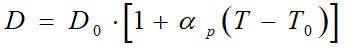2. Calculate correction factor for steam quality (For lliquid, water, and steam, Fs=1):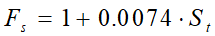3. Calculate orifice diameter at flow temperature.

1. For Venturi tubes and Nozzles: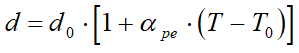2. For orifice plates: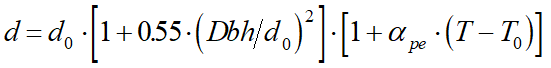4. Calculate b - ratio: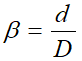5. Checking for Critical flow and some initial calculations.

Liquid /Water case

1. Calculate downstream pressure: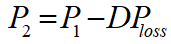2. If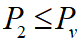the the flow is critical. If not then the floe is sub-critical.

1. For critical flow: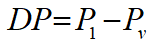2. For sub-critical flow:

Classic Venturi tube: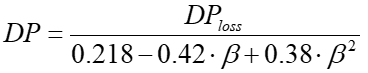1. Nozzles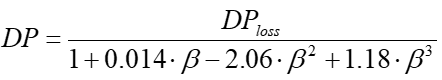2. Orifice plates: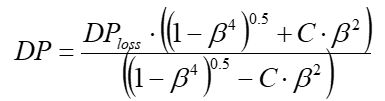3. Calculate first estimate for flow rate (W0 [kg/s]):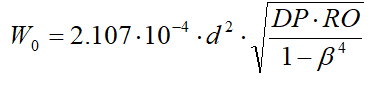3. Gas/Steam case

1. Calculate critical pressure ratio and define whether the flow is critical.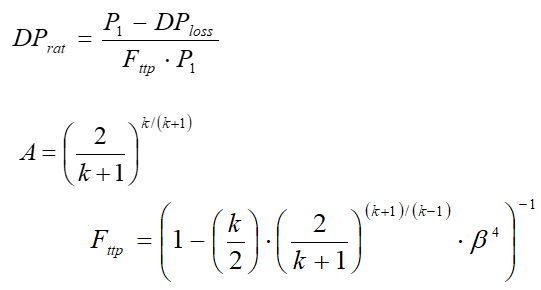If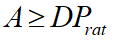the the flow is critical. If not the flow is sub-critical.

4. Initial calculation for critical flow.

1. Gas Expansion factor: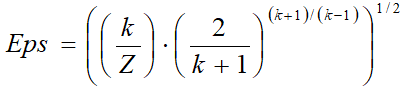2. First estimate for flow rate (W0 [1b/h], T[R], D[in], P1[psi-a])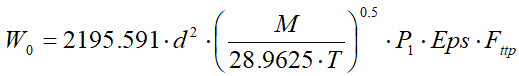5. Initial calculations for sub-critical flow.

Differential pressure to be used in flow rat calculation:

1. For classical Venturi tube: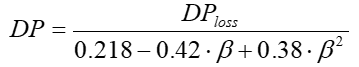2. For Nozzles: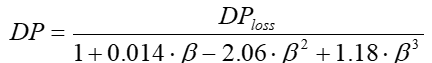3. For orifice plates: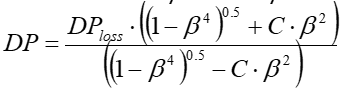6. Gas expansion factor:

1. For Venturi tubes and nozzles: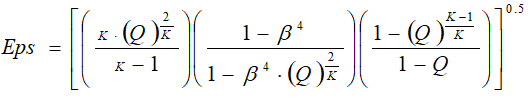where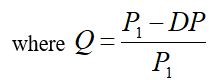2. For orifice plates: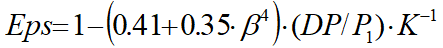3. First estimate for flow rate (W0[kg/s]):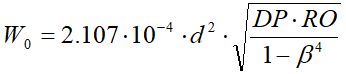6. Calculate Reynolds number.

1. For gas/steam critical flow (Bore Reynolds number):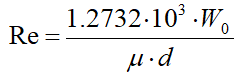2. For liquid/water and gas/steam sub-critical flow (Pipe Reynolds number):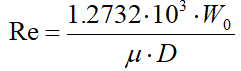7. Calculate discharge coefficient. See Appendix.

8. For orifices in the case of gas/steam sub-critical flow, calculate DP and gas expansion factor: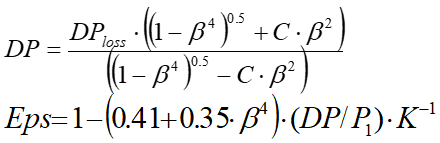9. Calculate new flow rate:

1. For gas/steam at critical flow: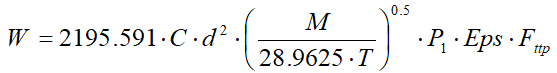2. For other cases (W[kg/s]):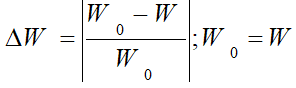10. Repeat steps 6 to 10 until the relative change in the flow rate is less than 0.0001.